# RD Sharma Solutions for Class 11 Maths Chapter 12 Mathematical Induction

## RD Sharma Solutions Class 11 Maths Chapter 12 – Download Free PDF Updated for 2021 – 22

RD Sharma Solutions for Class 11 Maths Chapter 12 – Mathematical Induction are provided here for students to score good marks in the board exams. Problems pertaining to principles of mathematical induction are dealt with in this chapter. The solutions to this chapter are formulated by our expert tutors to boost their confidence level among students in understanding the concepts and methods to solve problems in a shorter period.

RD Sharma Class 11 Maths Solutions help students who aim to secure a good academic score in the board exams. This chapter of RD Sharma Class 11 mainly focuses on the concept of mathematical induction. To know more about this topic students can download the RD Sharma Solutions for Class 11 Maths Chapter 12 from the below-mentioned links.

Chapter 12 – Mathematical Induction contains two exercises and the RD Sharma Solutions present in this page provide solutions to the questions present in each exercise. Now, let us have a look at the concepts discussed in this chapter.

• Mathematical statements.
• The principles of mathematical induction.
• The first principle of mathematical induction.
• The second principle of mathematical induction.

## Download the Pdf of RD Sharma Solutions for Class 11 Maths Chapter 12 – Mathematical Induction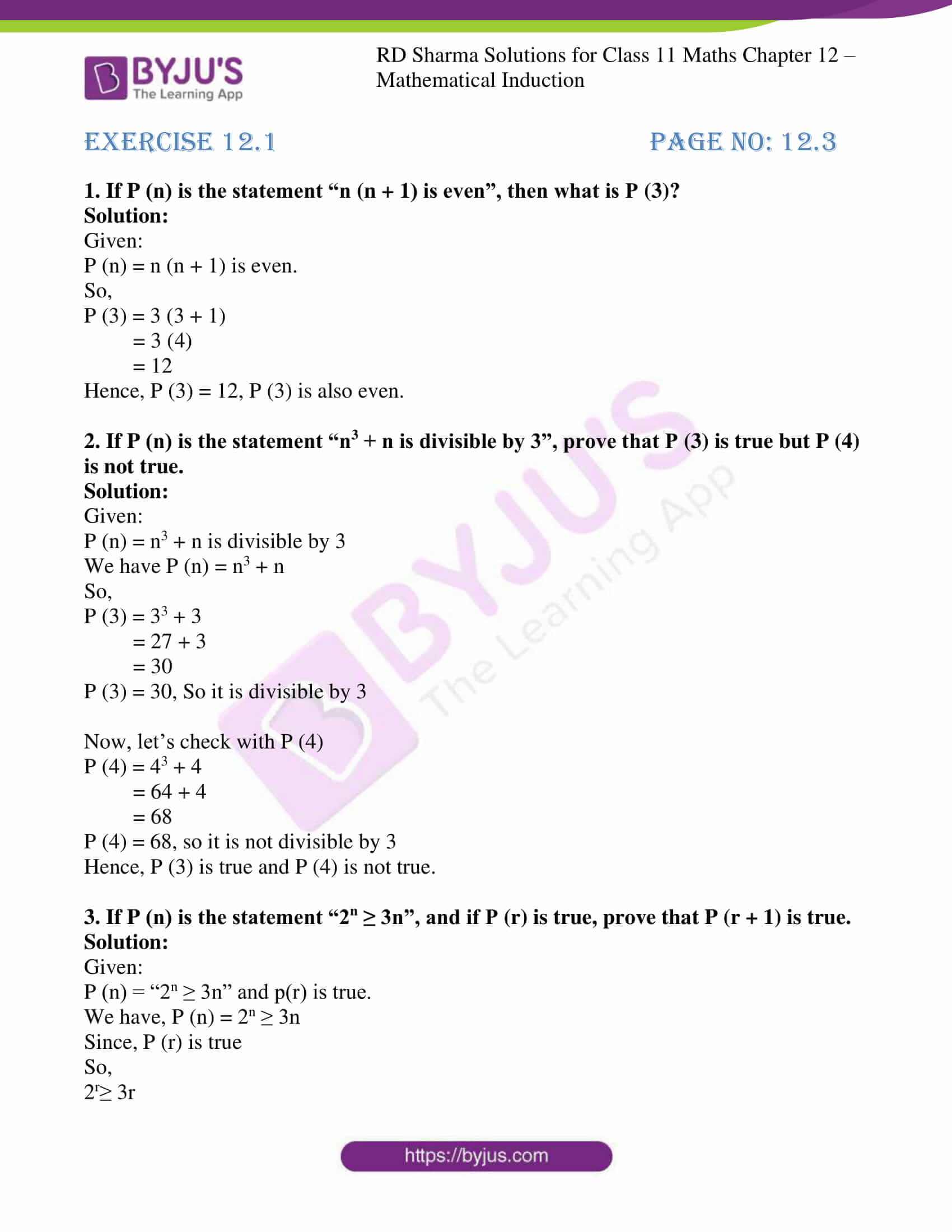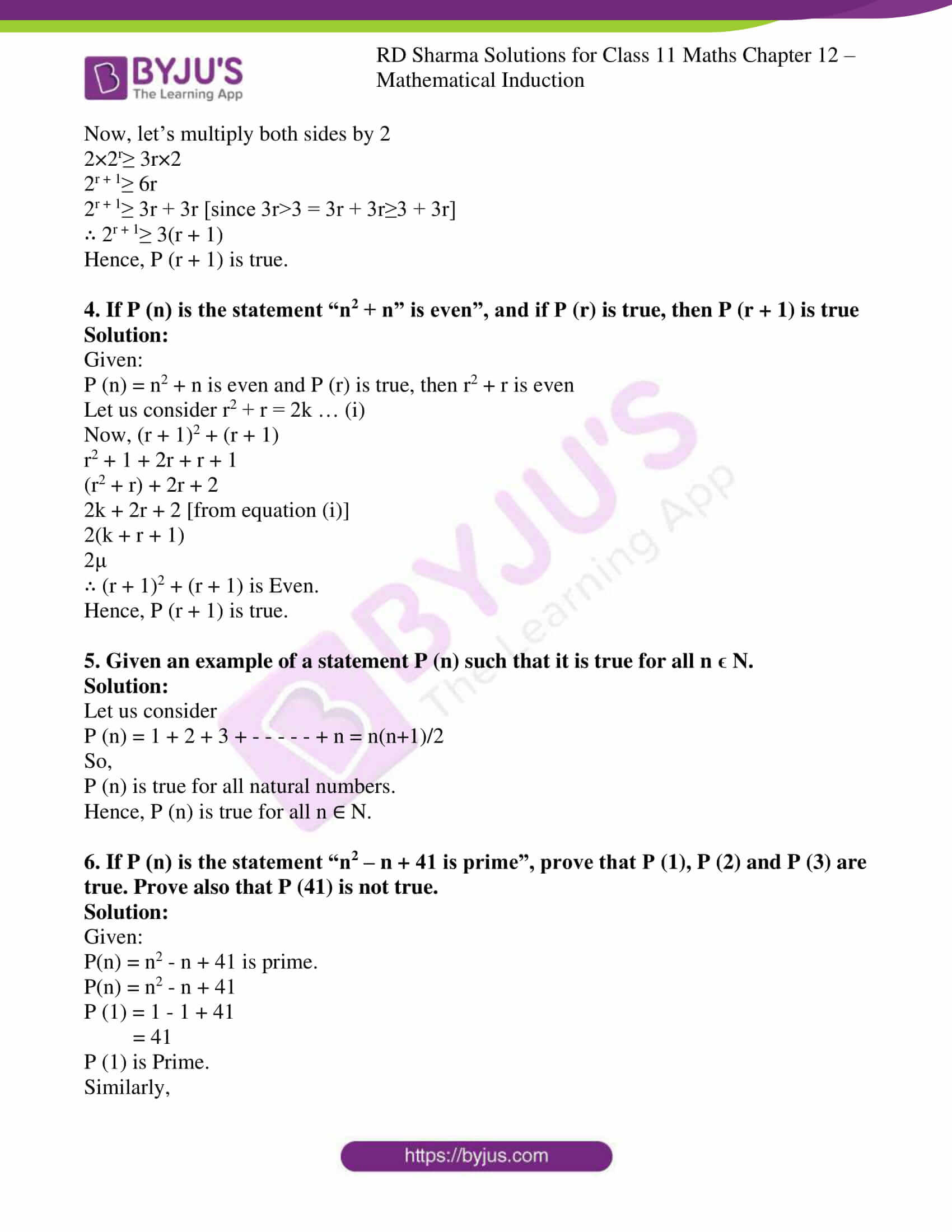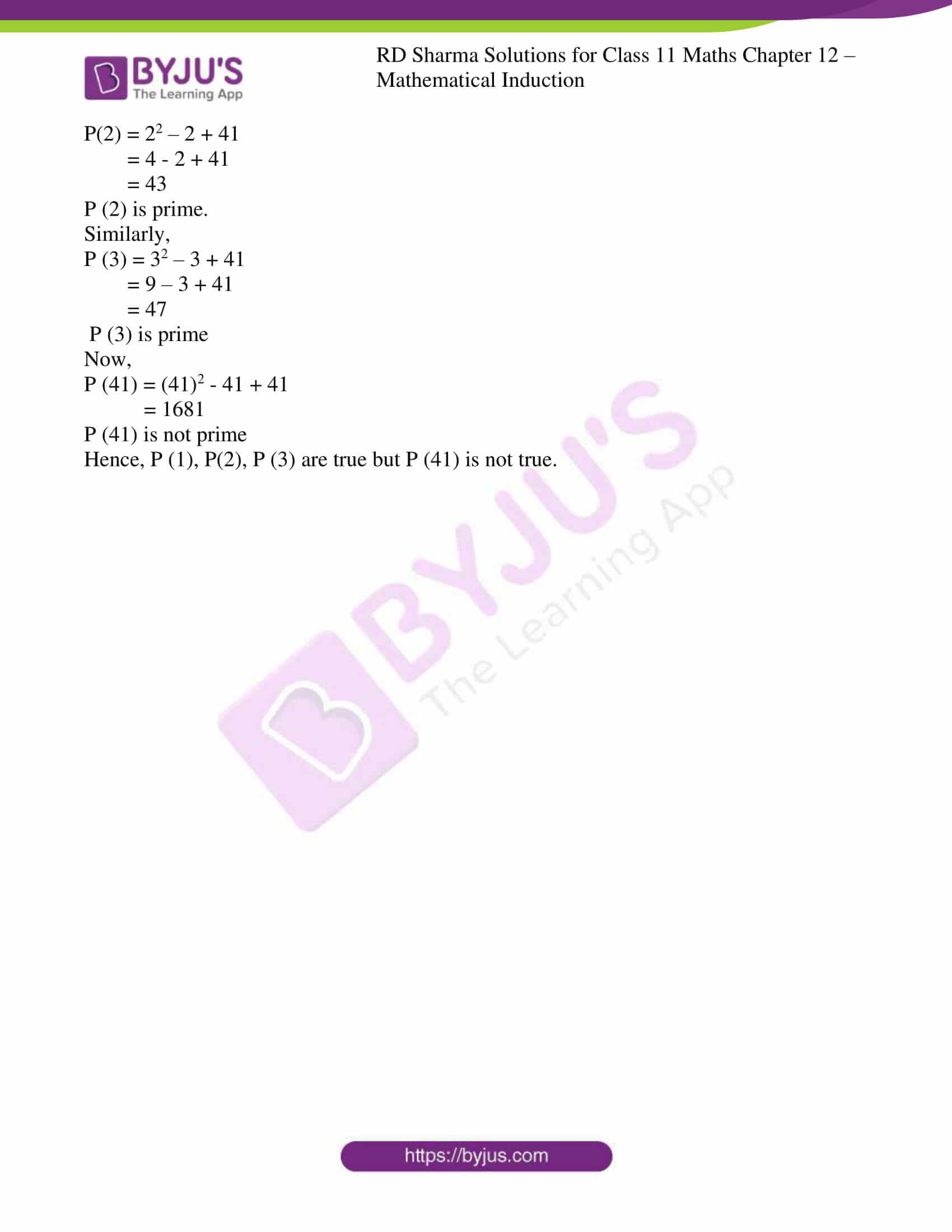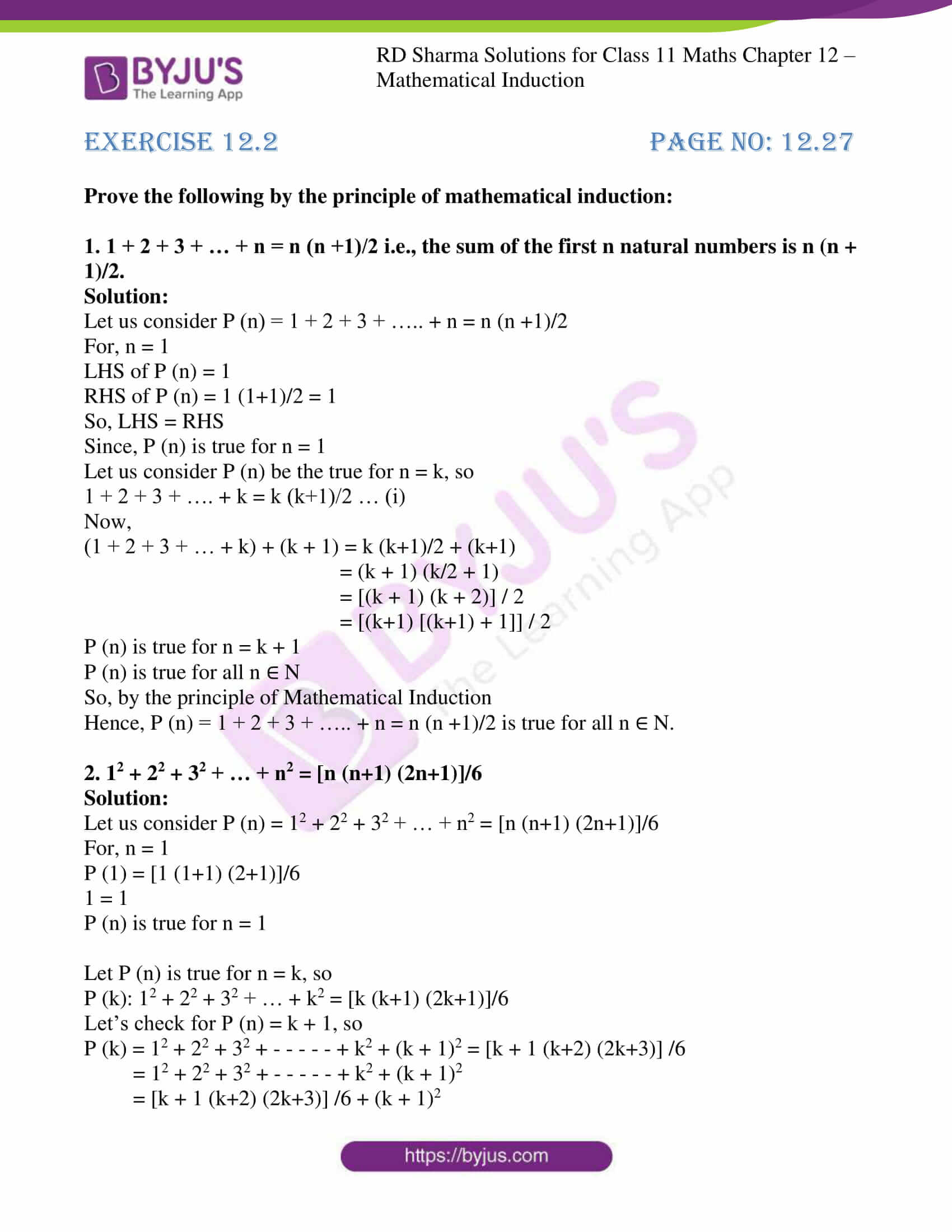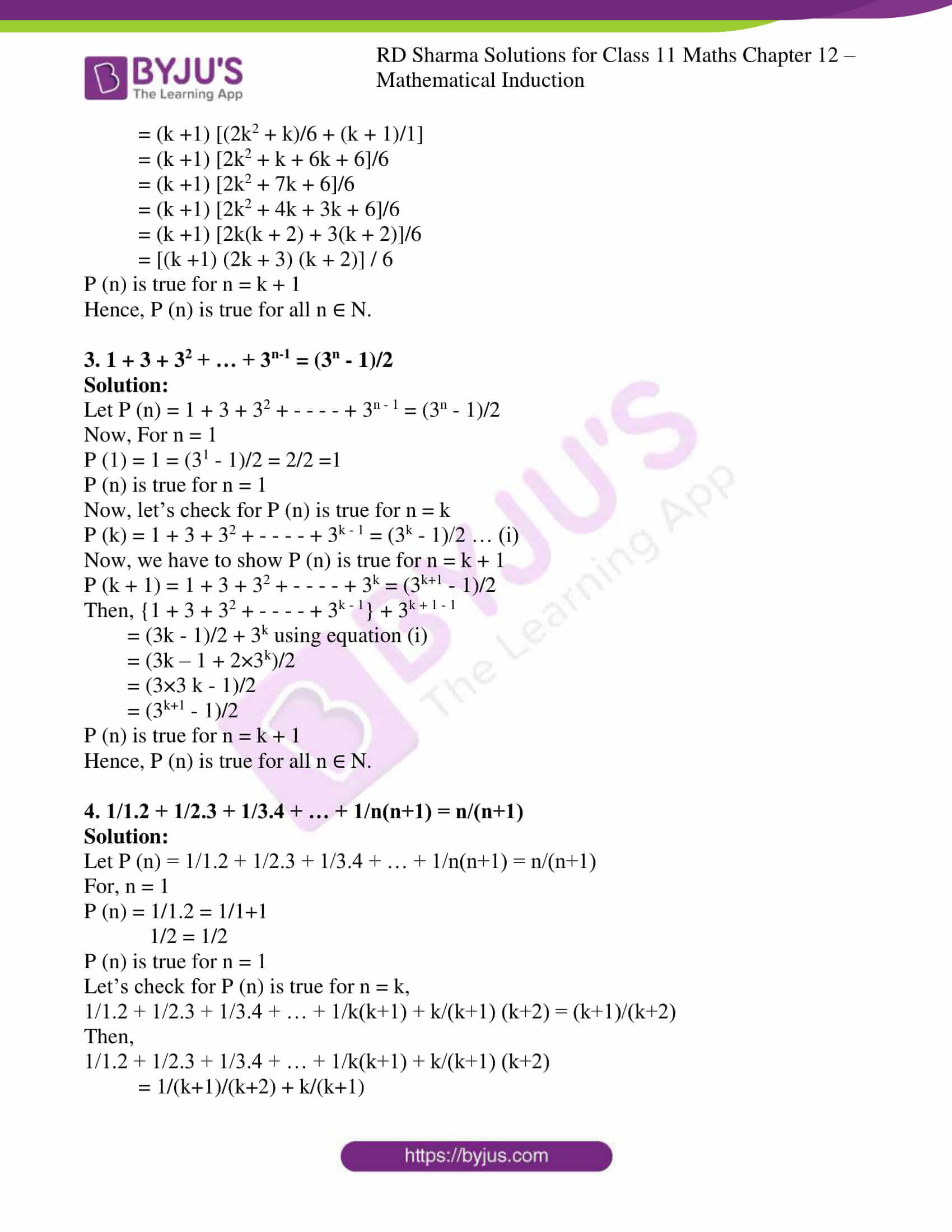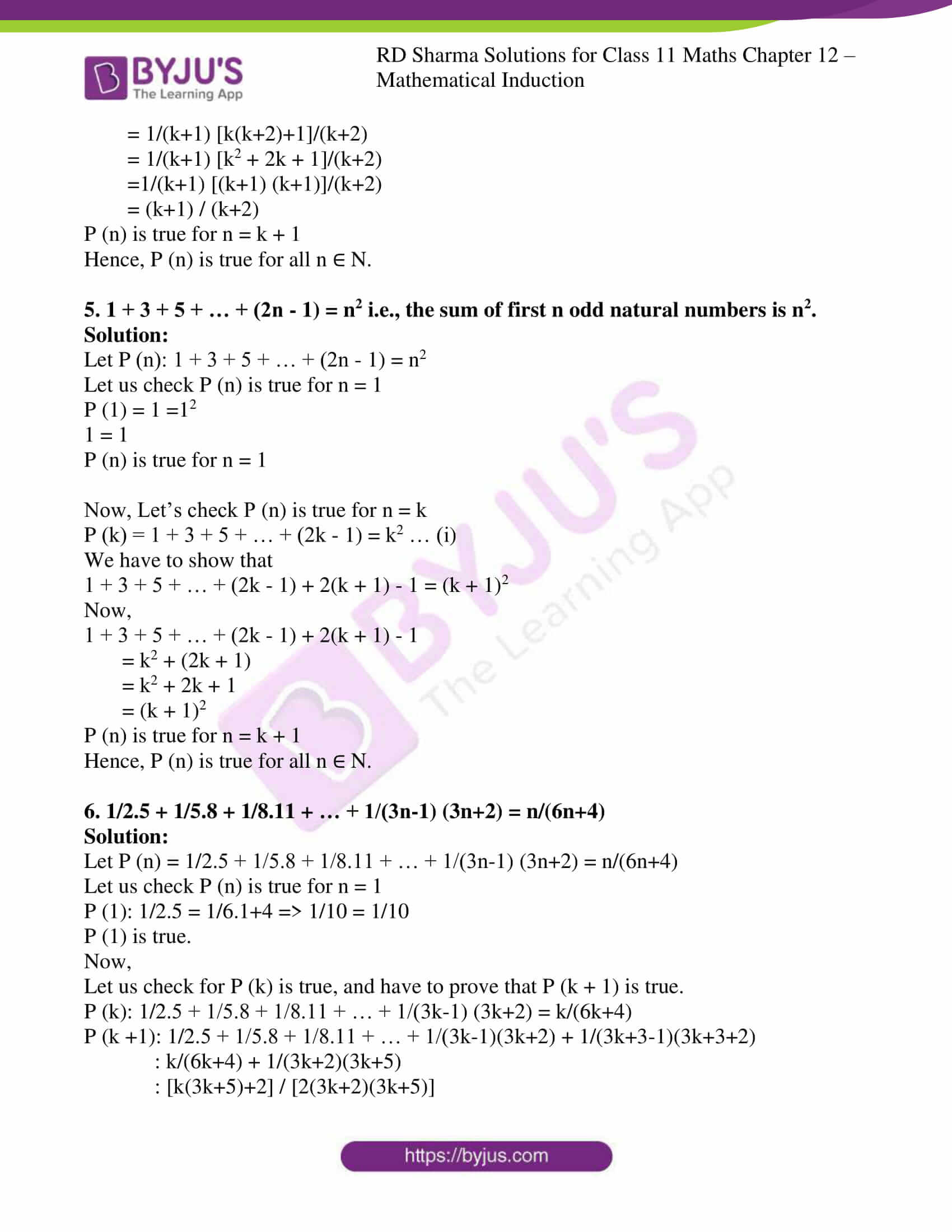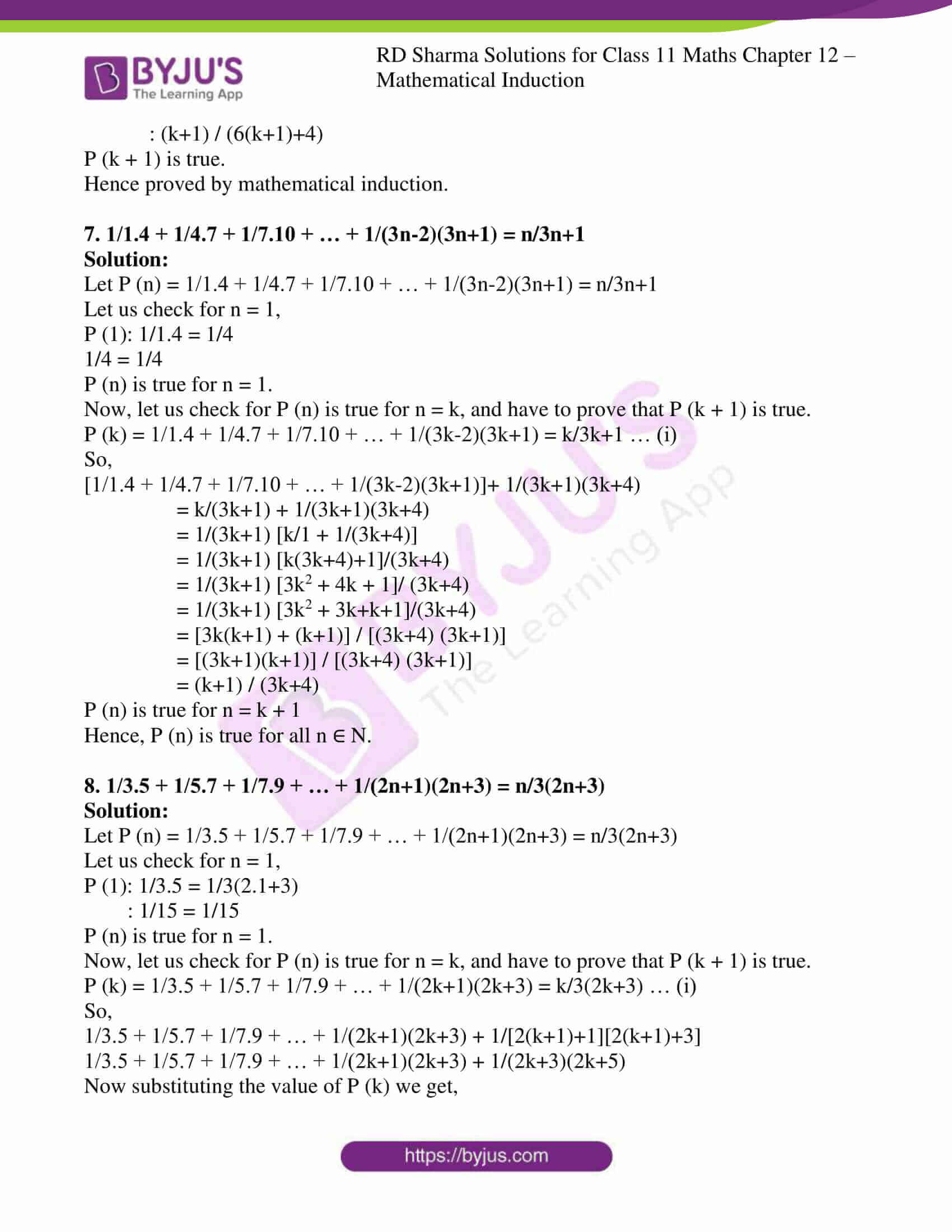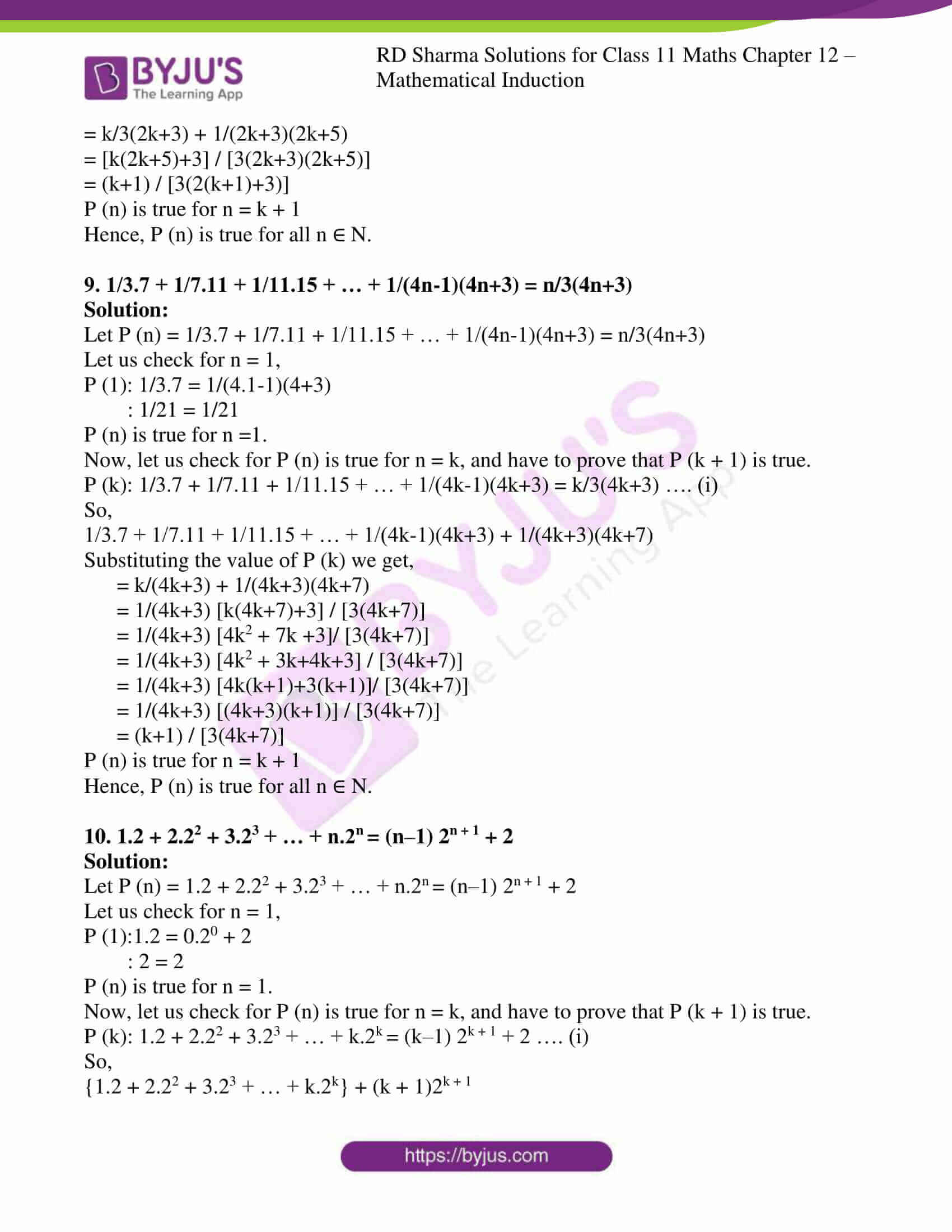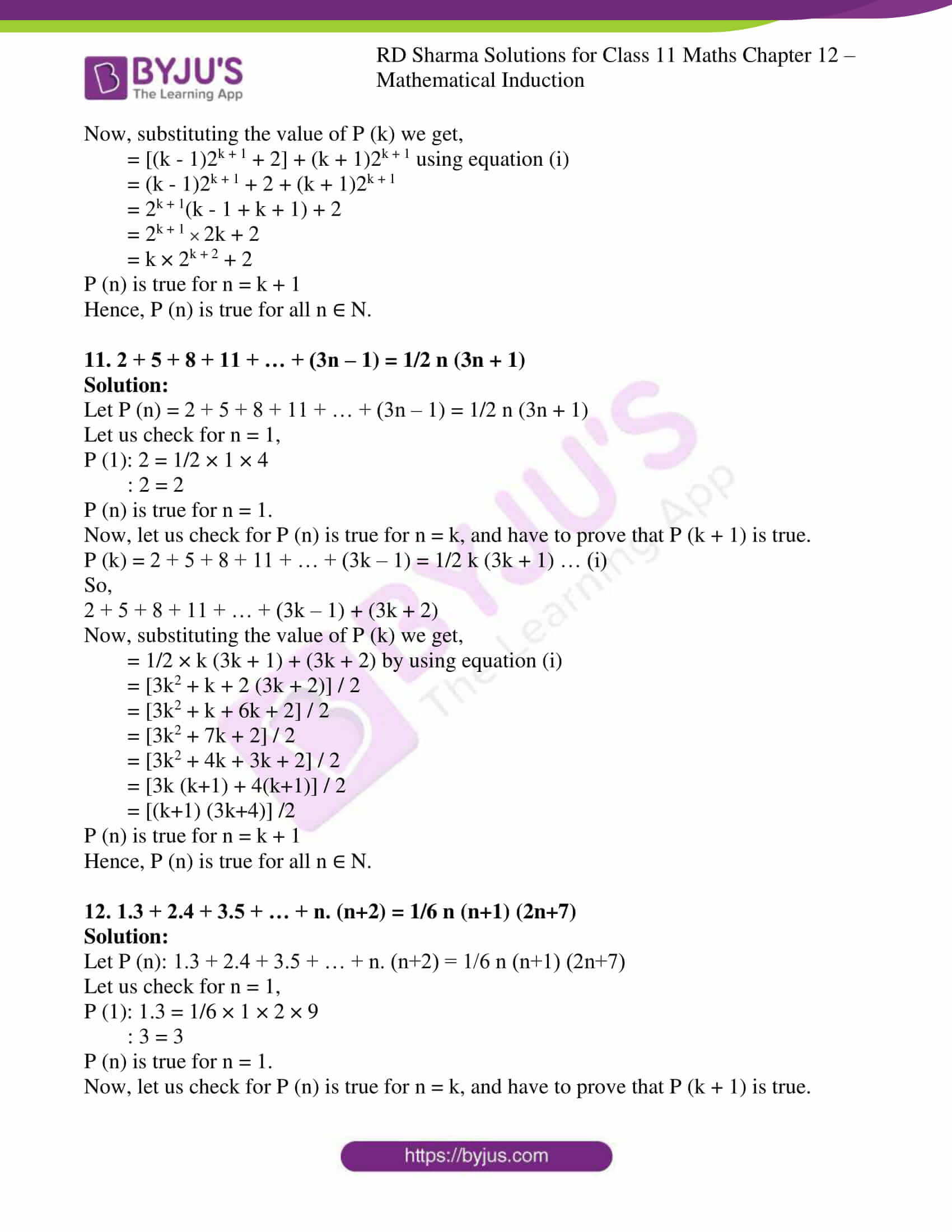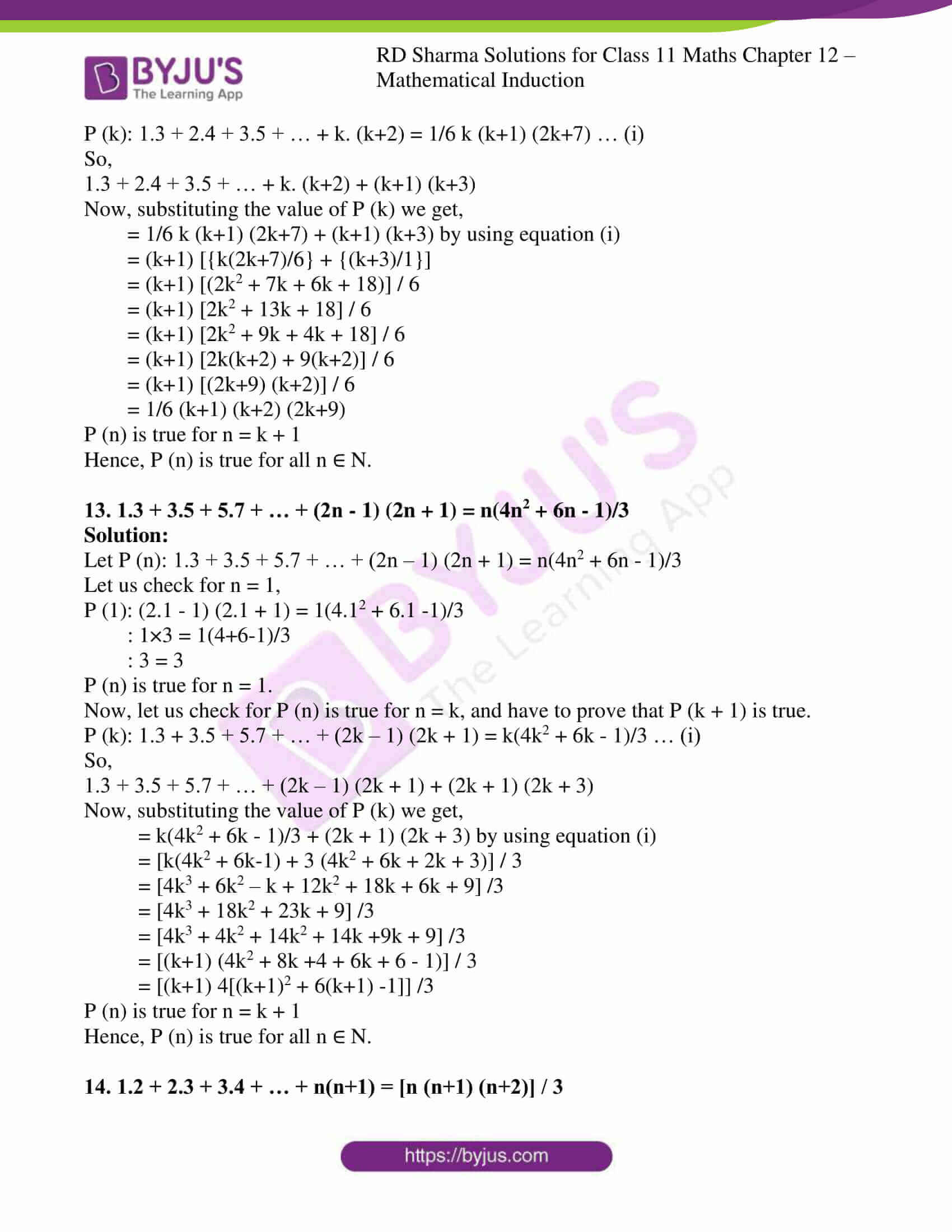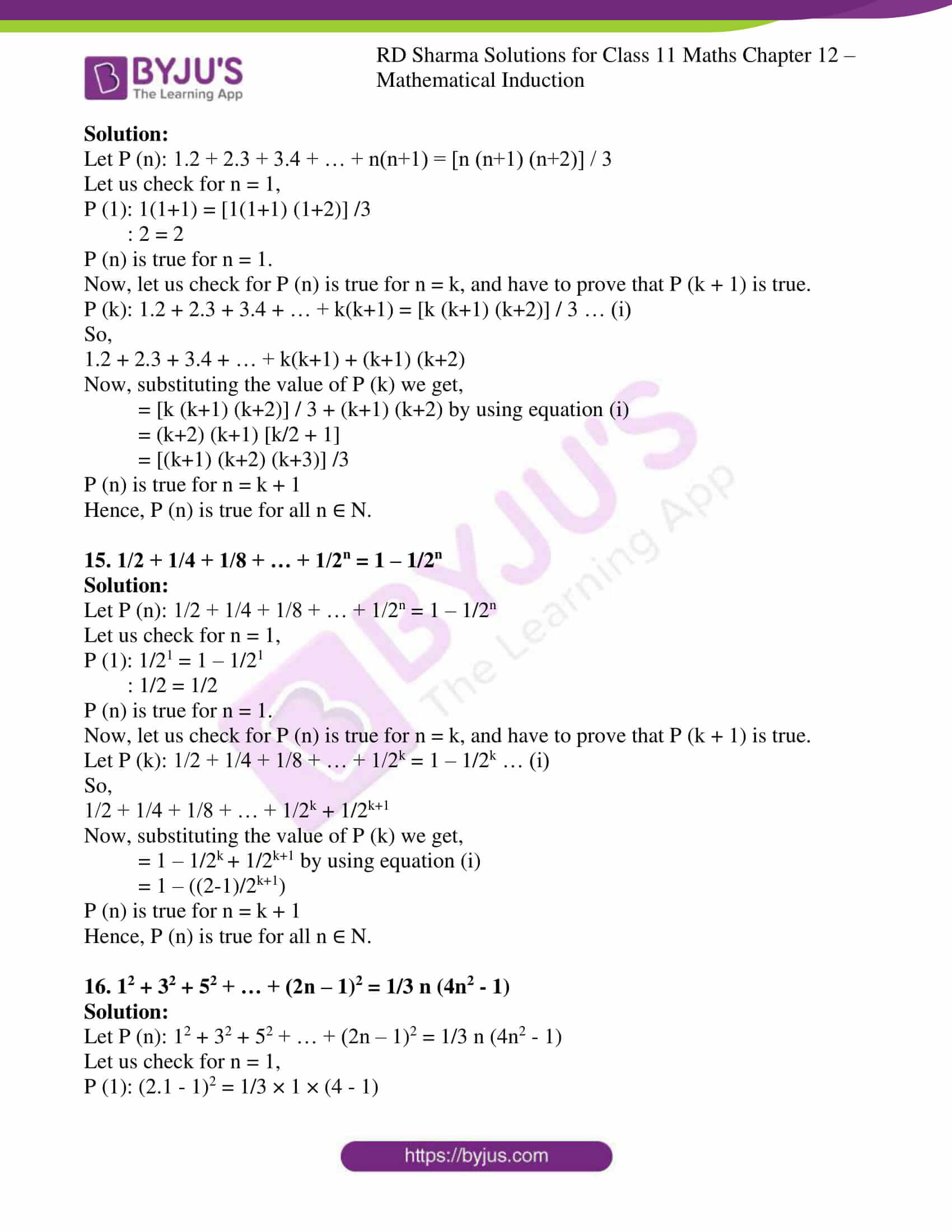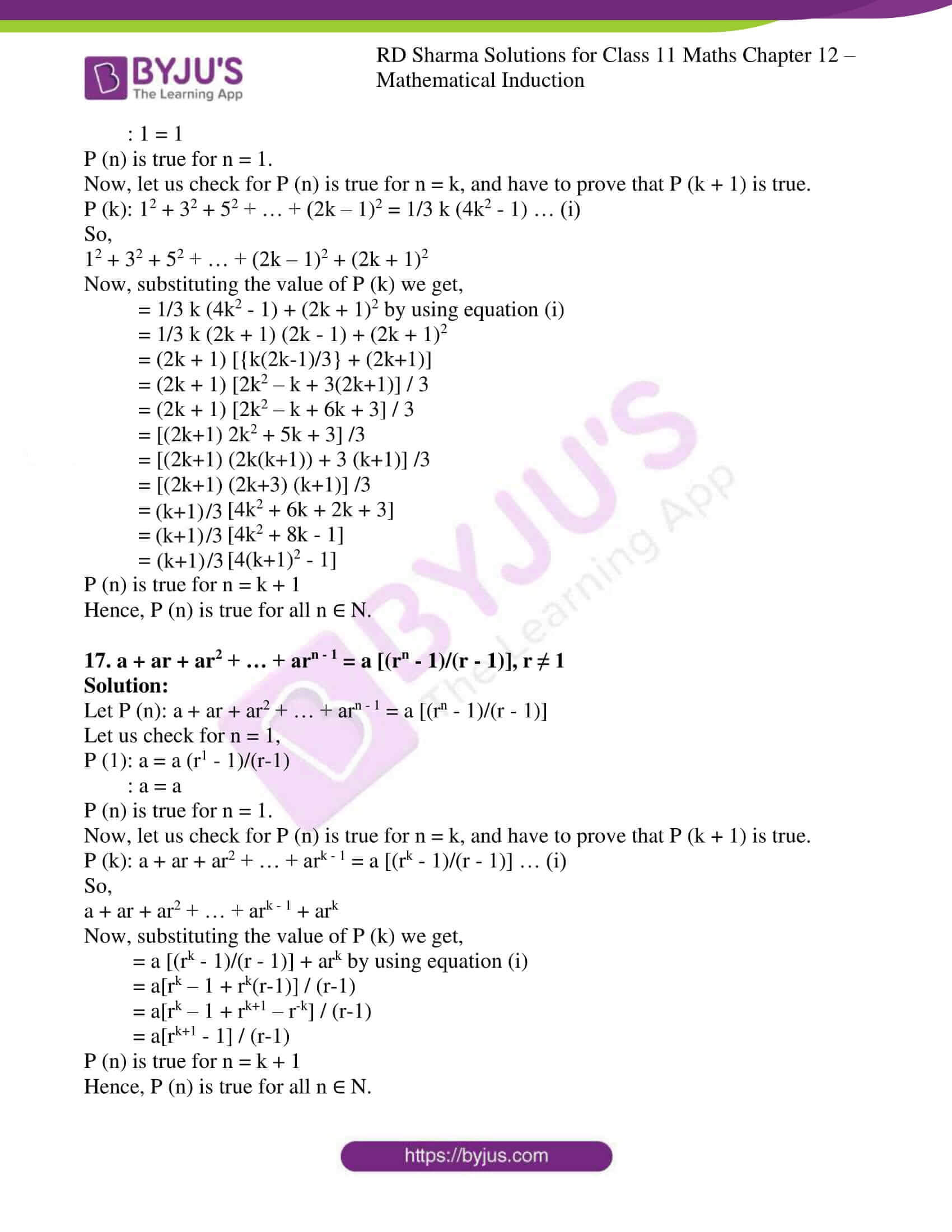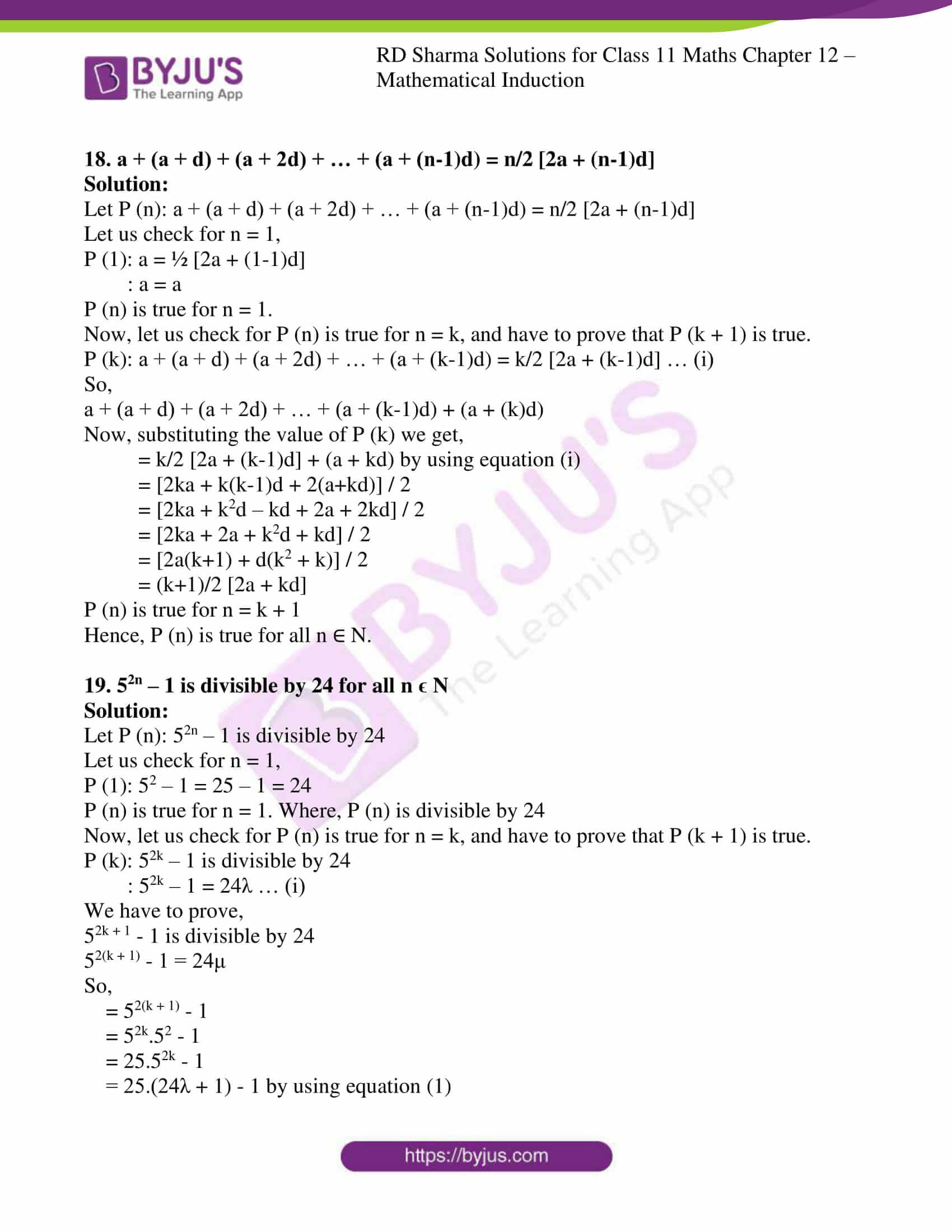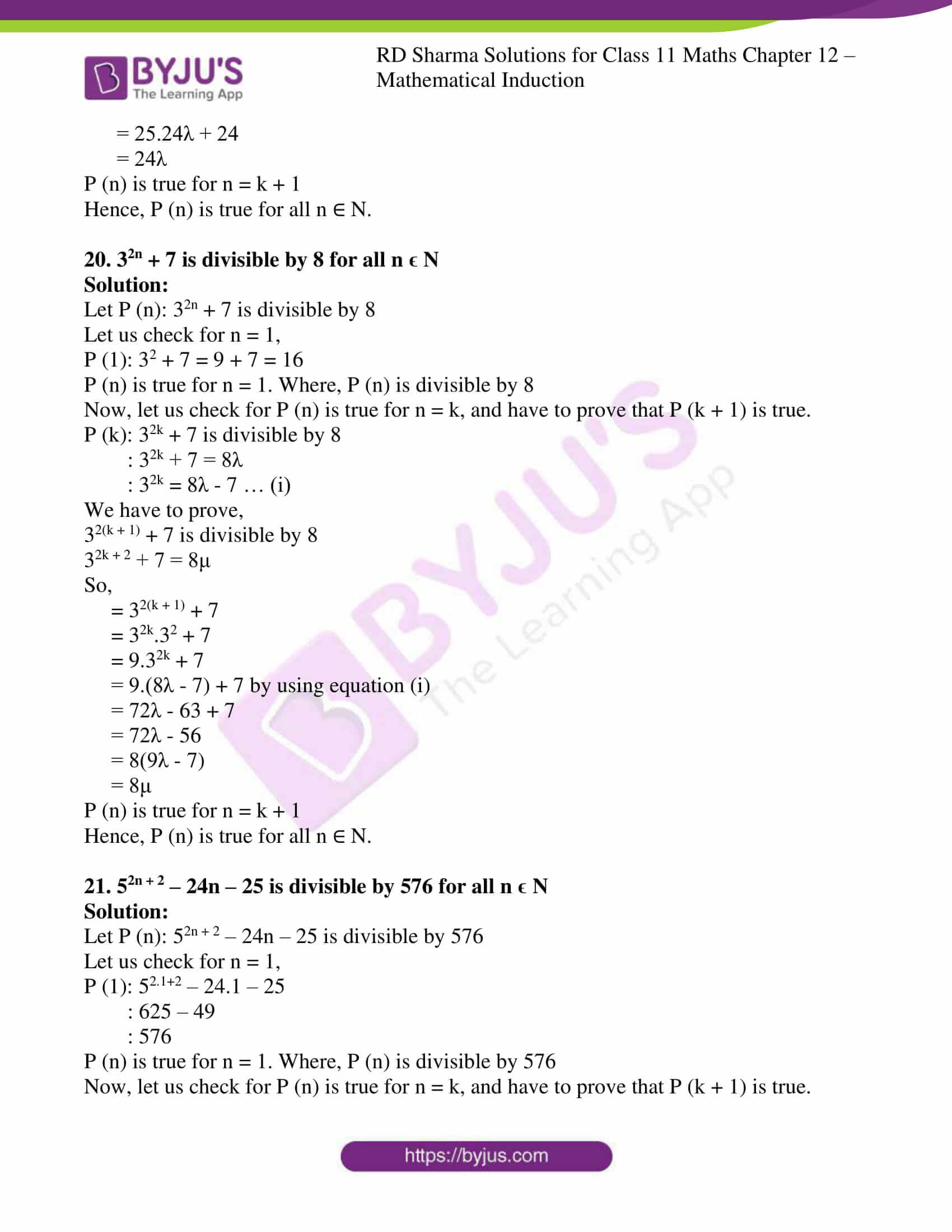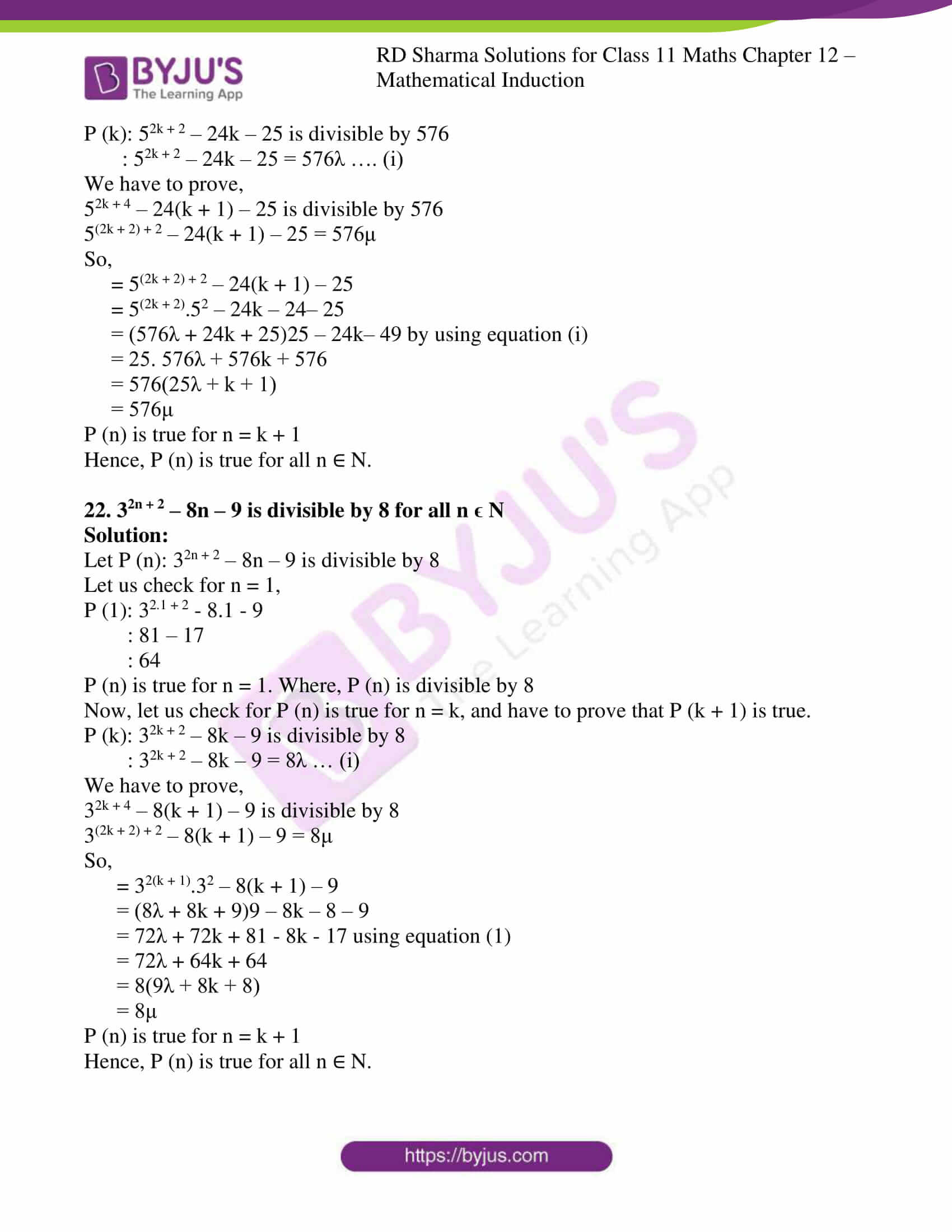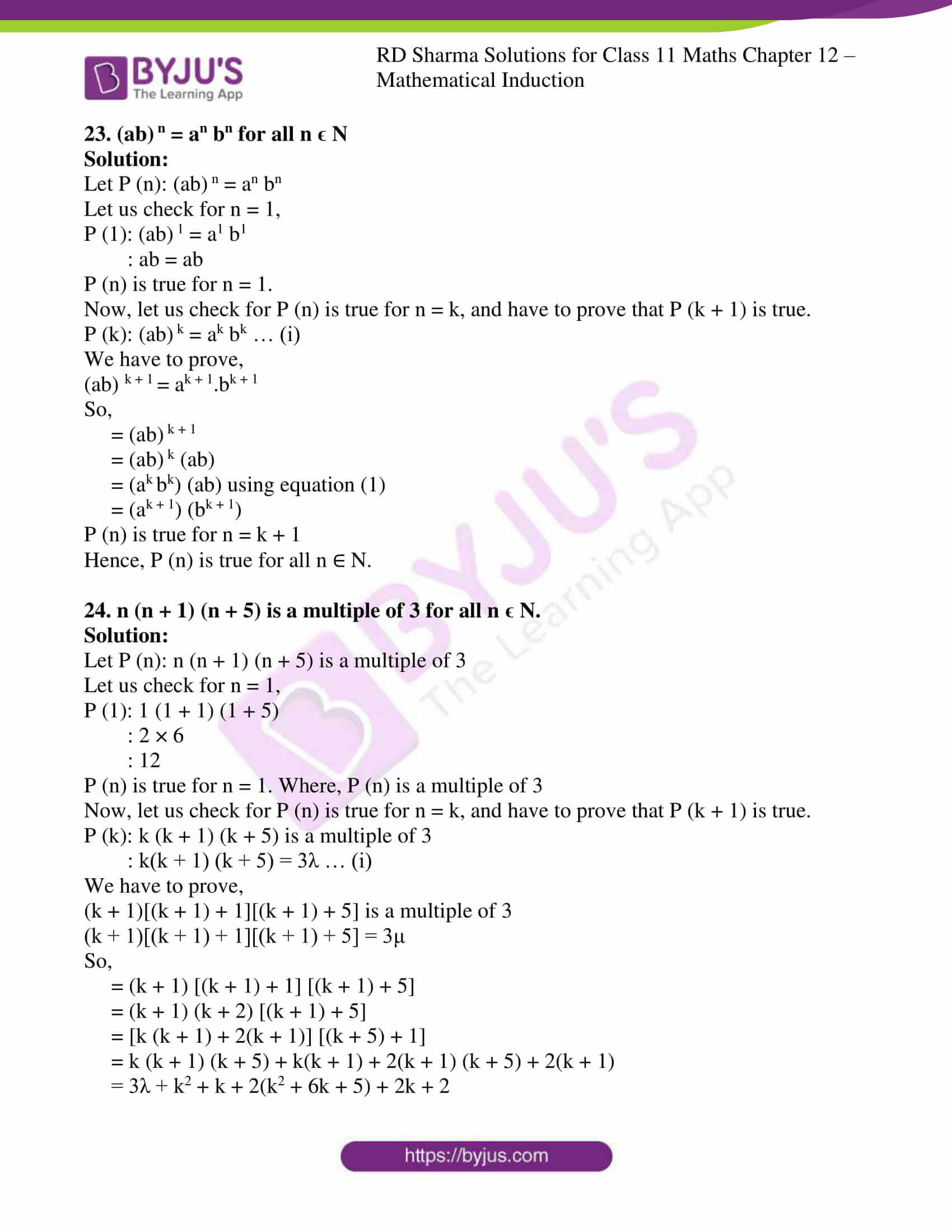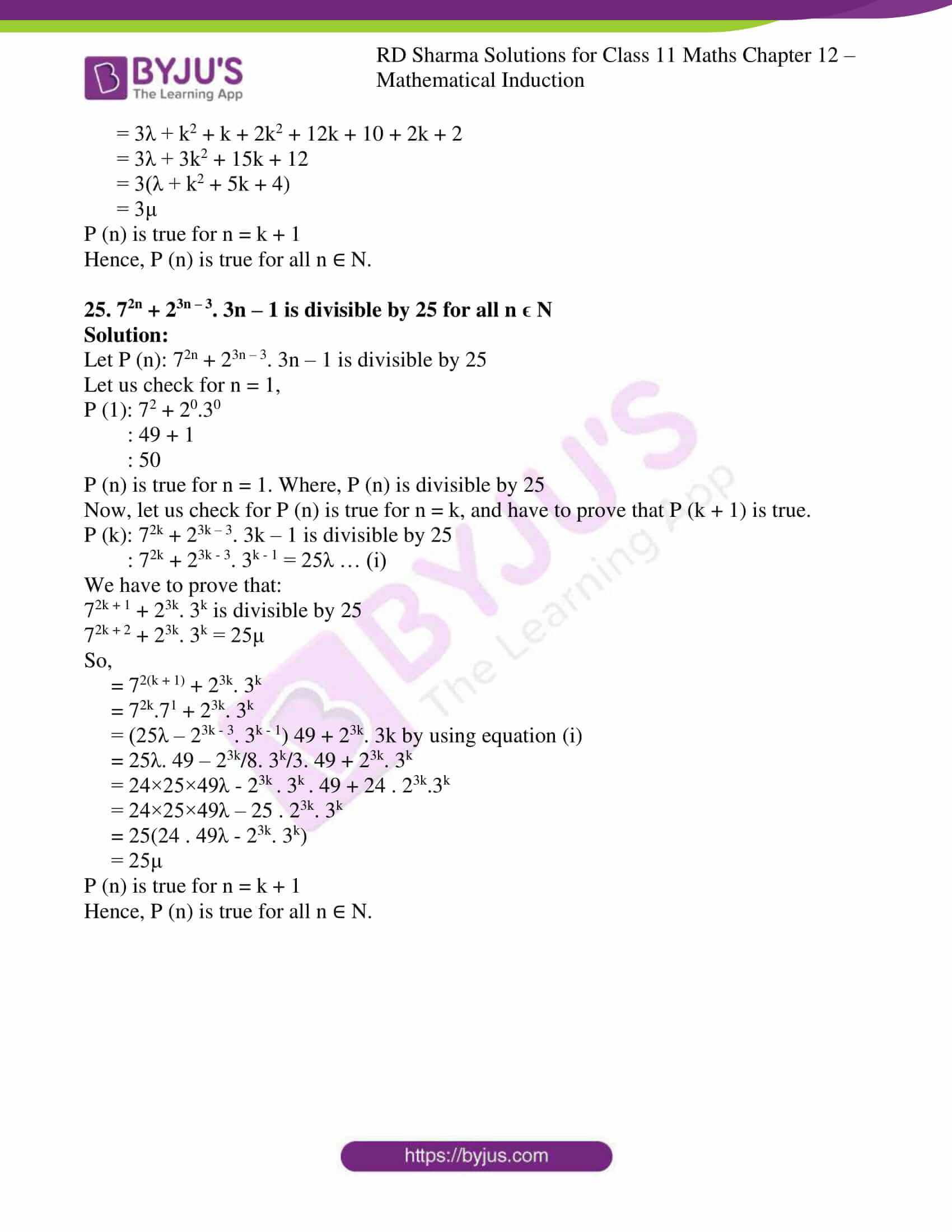### Also, access exercises of RD Sharma Solutions for Class 11 Maths Chapter 12 – Mathematical Induction

Exercise 12.1 Solutions

Exercise 12.2 Solutions

### Access answers to RD Sharma Solutions for Class 11 Maths Chapter 12 – Mathematical Induction

EXERCISE 12.1 PAGE NO: 12.3

1. If P (n) is the statement “n (n + 1) is even”, then what is P (3)?
Solution:

Given:

P (n) = n (n + 1) is even.

So,

P (3) = 3 (3 + 1)

= 3 (4)

= 12

Hence, P (3) = 12, P (3) is also even.

2. If P (n) is the statement “n3 + n is divisible by 3”, prove that P (3) is true but P (4) is not true.

Solution:

Given:

P (n) = n3 + n is divisible by 3

We have P (n) = n3 + n

So,

P (3) = 33 + 3

= 27 + 3

= 30

P (3) = 30, So it is divisible by 3

Now, let’s check with P (4)

P (4) = 43 + 4

= 64 + 4

= 68

P (4) = 68, so it is not divisible by 3

Hence, P (3) is true and P (4) is not true.

3. If P (n) is the statement “2n ≥ 3n”, and if P (r) is true, prove that P (r + 1) is true.

Solution:

Given:

P (n) = “2n ≥ 3n” and p(r) is true.

We have, P (n) = 2n ≥ 3n

Since, P (r) is true

So,

2r≥ 3r

Now, let’s multiply both sides by 2

2×2r≥ 3r×2

2r + 1≥ 6r

2r + 1≥ 3r + 3r [since 3r>3 = 3r + 3r≥3 + 3r]

∴ 2r + 1≥ 3(r + 1)

Hence, P (r + 1) is true.

4. If P (n) is the statement “n2 + n” is even”, and if P (r) is true, then P (r + 1) is true
Solution:

Given:

P (n) = n2 + n is even and P (r) is true, then r2 + r is even

Let us consider r2 + r = 2k … (i)

Now, (r + 1)2 + (r + 1)

r2 + 1 + 2r + r + 1

(r2 + r) + 2r + 2

2k + 2r + 2 [from equation (i)]

2(k + r + 1)

∴ (r + 1)2 + (r + 1) is Even.

Hence, P (r + 1) is true.

5. Given an example of a statement P (n) such that it is true for all n ϵ N.

Solution:

Let us consider

P (n) = 1 + 2 + 3 + – – – – – + n = n(n+1)/2

So,

P (n) is true for all natural numbers.

Hence, P (n) is true for all n ∈ N.

6. If P (n) is the statement “n2 – n + 41 is prime”, prove that P (1), P (2) and P (3) are true. Prove also that P (41) is not true.
Solution:

Given:

P(n) = n2 – n + 41 is prime.

P(n) = n2 – n + 41

P (1) = 1 – 1 + 41

= 41

P (1) is Prime.

Similarly,

P(2) = 22 – 2 + 41

= 4 – 2 + 41

= 43

P (2) is prime.

Similarly,

P (3) = 32 – 3 + 41

= 9 – 3 + 41

= 47

P (3) is prime

Now,

P (41) = (41)2 – 41 + 41

= 1681

P (41) is not prime

Hence, P (1), P(2), P (3) are true but P (41) is not true.

EXERCISE 12.2 PAGE NO: 12.27

Prove the following by the principle of mathematical induction:

1. 1 + 2 + 3 + … + n = n (n +1)/2 i.e., the sum of the first n natural numbers is n (n + 1)/2.

Solution:

Let us consider P (n) = 1 + 2 + 3 + ….. + n = n (n +1)/2

For, n = 1

LHS of P (n) = 1

RHS of P (n) = 1 (1+1)/2 = 1

So, LHS = RHS

Since, P (n) is true for n = 1

Let us consider P (n) be the true for n = k, so

1 + 2 + 3 + …. + k = k (k+1)/2 … (i)

Now,

(1 + 2 + 3 + … + k) + (k + 1) = k (k+1)/2 + (k+1)

= (k + 1) (k/2 + 1)

= [(k + 1) (k + 2)] / 2

= [(k+1) [(k+1) + 1]] / 2

P (n) is true for n = k + 1

P (n) is true for all n ∈ N

So, by the principle of Mathematical Induction

Hence, P (n) = 1 + 2 + 3 + ….. + n = n (n +1)/2 is true for all n ∈ N.

2. 12 + 22 + 32 + … + n2 = [n (n+1) (2n+1)]/6

Solution:

Let us consider P (n) = 12 + 22 + 32 + … + n2 = [n (n+1) (2n+1)]/6

For, n = 1

P (1) = [1 (1+1) (2+1)]/6

1 = 1

P (n) is true for n = 1

Let P (n) is true for n = k, so

P (k): 12 + 22 + 32 + … + k2 = [k (k+1) (2k+1)]/6

Let’s check for P (n) = k + 1, so

P (k) = 12 + 22 + 32 + – – – – – + k2 + (k + 1)2 = [k + 1 (k+2) (2k+3)] /6

= 12 + 22 + 32 + – – – – – + k2 + (k + 1)2

= [k + 1 (k+2) (2k+3)] /6 + (k + 1)2

= (k +1) [(2k2 + k)/6 + (k + 1)/1]

= (k +1) [2k2 + k + 6k + 6]/6

= (k +1) [2k2 + 7k + 6]/6

= (k +1) [2k2 + 4k + 3k + 6]/6

= (k +1) [2k(k + 2) + 3(k + 2)]/6

= [(k +1) (2k + 3) (k + 2)] / 6

P (n) is true for n = k + 1

Hence, P (n) is true for all n ∈ N.

3. 1 + 3 + 32 + … + 3n-1 = (3n – 1)/2

Solution:

Let P (n) = 1 + 3 + 32 + – – – – + 3n – 1 = (3n – 1)/2

Now, For n = 1

P (1) = 1 = (31 – 1)/2 = 2/2 =1

P (n) is true for n = 1

Now, let’s check for P (n) is true for n = k

P (k) = 1 + 3 + 32 + – – – – + 3k – 1 = (3k – 1)/2 … (i)

Now, we have to show P (n) is true for n = k + 1

P (k + 1) = 1 + 3 + 32 + – – – – + 3k = (3k+1 – 1)/2

Then, {1 + 3 + 32 + – – – – + 3k – 1} + 3k + 1 – 1

= (3k – 1)/2 + 3k using equation (i)

= (3k – 1 + 2×3k)/2

= (3×3 k – 1)/2

= (3k+1 – 1)/2

P (n) is true for n = k + 1

Hence, P (n) is true for all n ∈ N.

4. 1/1.2 + 1/2.3 + 1/3.4 + … + 1/n(n+1) = n/(n+1)

Solution:

Let P (n) = 1/1.2 + 1/2.3 + 1/3.4 + … + 1/n(n+1) = n/(n+1)

For, n = 1

P (n) = 1/1.2 = 1/1+1

1/2 = 1/2

P (n) is true for n = 1

Let’s check for P (n) is true for n = k,

1/1.2 + 1/2.3 + 1/3.4 + … + 1/k(k+1) + k/(k+1) (k+2) = (k+1)/(k+2)

Then,

1/1.2 + 1/2.3 + 1/3.4 + … + 1/k(k+1) + k/(k+1) (k+2)

= 1/(k+1)/(k+2) + k/(k+1)

= 1/(k+1) [k(k+2)+1]/(k+2)

= 1/(k+1) [k2 + 2k + 1]/(k+2)

=1/(k+1) [(k+1) (k+1)]/(k+2)

= (k+1) / (k+2)

P (n) is true for n = k + 1

Hence, P (n) is true for all n ∈ N.

5. 1 + 3 + 5 + … + (2n – 1) = n2 i.e., the sum of first n odd natural numbers is n2.

Solution:

Let P (n): 1 + 3 + 5 + … + (2n – 1) = n2

Let us check P (n) is true for n = 1

P (1) = 1 =12

1 = 1

P (n) is true for n = 1

Now, Let’s check P (n) is true for n = k

P (k) = 1 + 3 + 5 + … + (2k – 1) = k2 … (i)

We have to show that

1 + 3 + 5 + … + (2k – 1) + 2(k + 1) – 1 = (k + 1)2

Now,

1 + 3 + 5 + … + (2k – 1) + 2(k + 1) – 1

= k2 + (2k + 1)

= k2 + 2k + 1

= (k + 1)2

P (n) is true for n = k + 1

Hence, P (n) is true for all n ∈ N.

6. 1/2.5 + 1/5.8 + 1/8.11 + … + 1/(3n-1) (3n+2) = n/(6n+4)

Solution:

Let P (n) = 1/2.5 + 1/5.8 + 1/8.11 + … + 1/(3n-1) (3n+2) = n/(6n+4)

Let us check P (n) is true for n = 1

P (1): 1/2.5 = 1/6.1+4 => 1/10 = 1/10

P (1) is true.

Now,

Let us check for P (k) is true, and have to prove that P (k + 1) is true.

P (k): 1/2.5 + 1/5.8 + 1/8.11 + … + 1/(3k-1) (3k+2) = k/(6k+4)

P (k +1): 1/2.5 + 1/5.8 + 1/8.11 + … + 1/(3k-1)(3k+2) + 1/(3k+3-1)(3k+3+2)

: k/(6k+4) + 1/(3k+2)(3k+5)

: [k(3k+5)+2] / [2(3k+2)(3k+5)]

: (k+1) / (6(k+1)+4)

P (k + 1) is true.

Hence proved by mathematical induction.

7. 1/1.4 + 1/4.7 + 1/7.10 + … + 1/(3n-2)(3n+1) = n/3n+1

Solution:

Let P (n) = 1/1.4 + 1/4.7 + 1/7.10 + … + 1/(3n-2)(3n+1) = n/3n+1

Let us check for n = 1,

P (1): 1/1.4 = 1/4

1/4 = 1/4

P (n) is true for n = 1.

Now, let us check for P (n) is true for n = k, and have to prove that P (k + 1) is true.

P (k) = 1/1.4 + 1/4.7 + 1/7.10 + … + 1/(3k-2)(3k+1) = k/3k+1 … (i)

So,

[1/1.4 + 1/4.7 + 1/7.10 + … + 1/(3k-2)(3k+1)]+ 1/(3k+1)(3k+4)

= k/(3k+1) + 1/(3k+1)(3k+4)

= 1/(3k+1) [k/1 + 1/(3k+4)]

= 1/(3k+1) [k(3k+4)+1]/(3k+4)

= 1/(3k+1) [3k2 + 4k + 1]/ (3k+4)

= 1/(3k+1) [3k2 + 3k+k+1]/(3k+4)

= [3k(k+1) + (k+1)] / [(3k+4) (3k+1)]

= [(3k+1)(k+1)] / [(3k+4) (3k+1)]

= (k+1) / (3k+4)

P (n) is true for n = k + 1

Hence, P (n) is true for all n ∈ N.

8. 1/3.5 + 1/5.7 + 1/7.9 + … + 1/(2n+1)(2n+3) = n/3(2n+3)

Solution:

Let P (n) = 1/3.5 + 1/5.7 + 1/7.9 + … + 1/(2n+1)(2n+3) = n/3(2n+3)

Let us check for n = 1,

P (1): 1/3.5 = 1/3(2.1+3)

: 1/15 = 1/15

P (n) is true for n = 1.

Now, let us check for P (n) is true for n = k, and have to prove that P (k + 1) is true.

P (k) = 1/3.5 + 1/5.7 + 1/7.9 + … + 1/(2k+1)(2k+3) = k/3(2k+3) … (i)

So,

1/3.5 + 1/5.7 + 1/7.9 + … + 1/(2k+1)(2k+3) + 1/[2(k+1)+1][2(k+1)+3]

1/3.5 + 1/5.7 + 1/7.9 + … + 1/(2k+1)(2k+3) + 1/(2k+3)(2k+5)

Now substituting the value of P (k) we get,

= k/3(2k+3) + 1/(2k+3)(2k+5)

= [k(2k+5)+3] / [3(2k+3)(2k+5)]

= (k+1) / [3(2(k+1)+3)]

P (n) is true for n = k + 1

Hence, P (n) is true for all n ∈ N.

9. 1/3.7 + 1/7.11 + 1/11.15 + … + 1/(4n-1)(4n+3) = n/3(4n+3)

Solution:

Let P (n) = 1/3.7 + 1/7.11 + 1/11.15 + … + 1/(4n-1)(4n+3) = n/3(4n+3)

Let us check for n = 1,

P (1): 1/3.7 = 1/(4.1-1)(4+3)

: 1/21 = 1/21

P (n) is true for n =1.

Now, let us check for P (n) is true for n = k, and have to prove that P (k + 1) is true.

P (k): 1/3.7 + 1/7.11 + 1/11.15 + … + 1/(4k-1)(4k+3) = k/3(4k+3) …. (i)

So,

1/3.7 + 1/7.11 + 1/11.15 + … + 1/(4k-1)(4k+3) + 1/(4k+3)(4k+7)

Substituting the value of P (k) we get,

= k/(4k+3) + 1/(4k+3)(4k+7)

= 1/(4k+3) [k(4k+7)+3] / [3(4k+7)]

= 1/(4k+3) [4k2 + 7k +3]/ [3(4k+7)]

= 1/(4k+3) [4k2 + 3k+4k+3] / [3(4k+7)]

= 1/(4k+3) [4k(k+1)+3(k+1)]/ [3(4k+7)]

= 1/(4k+3) [(4k+3)(k+1)] / [3(4k+7)]

= (k+1) / [3(4k+7)]

P (n) is true for n = k + 1

Hence, P (n) is true for all n ∈ N.

10. 1.2 + 2.22 + 3.23 + … + n.2n = (n–1) 2n + 1 + 2

Solution:

Let P (n) = 1.2 + 2.22 + 3.23 + … + n.2n = (n–1) 2n + 1 + 2

Let us check for n = 1,

P (1):1.2 = 0.20 + 2

: 2 = 2

P (n) is true for n = 1.

Now, let us check for P (n) is true for n = k, and have to prove that P (k + 1) is true.

P (k): 1.2 + 2.22 + 3.23 + … + k.2k = (k–1) 2k + 1 + 2 …. (i)

So,

{1.2 + 2.22 + 3.23 + … + k.2k} + (k + 1)2k + 1

Now, substituting the value of P (k) we get,

= [(k – 1)2k + 1 + 2] + (k + 1)2k + 1 using equation (i)

= (k – 1)2k + 1 + 2 + (k + 1)2k + 1

= 2k + 1(k – 1 + k + 1) + 2

= 2k + 1 × 2k + 2

= k × 2k + 2 + 2

P (n) is true for n = k + 1

Hence, P (n) is true for all n ∈ N.

11. 2 + 5 + 8 + 11 + … + (3n – 1) = 1/2 n (3n + 1)

Solution:

Let P (n) = 2 + 5 + 8 + 11 + … + (3n – 1) = 1/2 n (3n + 1)

Let us check for n = 1,

P (1): 2 = 1/2 × 1 × 4

: 2 = 2

P (n) is true for n = 1.

Now, let us check for P (n) is true for n = k, and have to prove that P (k + 1) is true.

P (k) = 2 + 5 + 8 + 11 + … + (3k – 1) = 1/2 k (3k + 1) … (i)

So,

2 + 5 + 8 + 11 + … + (3k – 1) + (3k + 2)

Now, substituting the value of P (k) we get,

= 1/2 × k (3k + 1) + (3k + 2) by using equation (i)

= [3k2 + k + 2 (3k + 2)] / 2

= [3k2 + k + 6k + 2] / 2

= [3k2 + 7k + 2] / 2

= [3k2 + 4k + 3k + 2] / 2

= [3k (k+1) + 4(k+1)] / 2

= [(k+1) (3k+4)] /2

P (n) is true for n = k + 1

Hence, P (n) is true for all n ∈ N.

12. 1.3 + 2.4 + 3.5 + … + n. (n+2) = 1/6 n (n+1) (2n+7)

Solution:

Let P (n): 1.3 + 2.4 + 3.5 + … + n. (n+2) = 1/6 n (n+1) (2n+7)

Let us check for n = 1,

P (1): 1.3 = 1/6 × 1 × 2 × 9

: 3 = 3

P (n) is true for n = 1.

Now, let us check for P (n) is true for n = k, and have to prove that P (k + 1) is true.

P (k): 1.3 + 2.4 + 3.5 + … + k. (k+2) = 1/6 k (k+1) (2k+7) … (i)

So,

1.3 + 2.4 + 3.5 + … + k. (k+2) + (k+1) (k+3)

Now, substituting the value of P (k) we get,

= 1/6 k (k+1) (2k+7) + (k+1) (k+3) by using equation (i)

= (k+1) [{k(2k+7)/6} + {(k+3)/1}]

= (k+1) [(2k2 + 7k + 6k + 18)] / 6

= (k+1) [2k2 + 13k + 18] / 6

= (k+1) [2k2 + 9k + 4k + 18] / 6

= (k+1) [2k(k+2) + 9(k+2)] / 6

= (k+1) [(2k+9) (k+2)] / 6

= 1/6 (k+1) (k+2) (2k+9)

P (n) is true for n = k + 1

Hence, P (n) is true for all n ∈ N.

13. 1.3 + 3.5 + 5.7 + … + (2n – 1) (2n + 1) = n(4n2 + 6n – 1)/3

Solution:

Let P (n): 1.3 + 3.5 + 5.7 + … + (2n – 1) (2n + 1) = n(4n2 + 6n – 1)/3

Let us check for n = 1,

P (1): (2.1 – 1) (2.1 + 1) = 1(4.12 + 6.1 -1)/3

: 1×3 = 1(4+6-1)/3

: 3 = 3

P (n) is true for n = 1.

Now, let us check for P (n) is true for n = k, and have to prove that P (k + 1) is true.

P (k): 1.3 + 3.5 + 5.7 + … + (2k – 1) (2k + 1) = k(4k2 + 6k – 1)/3 … (i)

So,

1.3 + 3.5 + 5.7 + … + (2k – 1) (2k + 1) + (2k + 1) (2k + 3)

Now, substituting the value of P (k) we get,

= k(4k2 + 6k – 1)/3 + (2k + 1) (2k + 3) by using equation (i)

= [k(4k2 + 6k-1) + 3 (4k2 + 6k + 2k + 3)] / 3

= [4k3 + 6k2 – k + 12k2 + 18k + 6k + 9] /3

= [4k3 + 18k2 + 23k + 9] /3

= [4k3 + 4k2 + 14k2 + 14k +9k + 9] /3

= [(k+1) (4k2 + 8k +4 + 6k + 6 – 1)] / 3

= [(k+1) 4[(k+1)2 + 6(k+1) -1]] /3

P (n) is true for n = k + 1

Hence, P (n) is true for all n ∈ N.

14. 1.2 + 2.3 + 3.4 + … + n(n+1) = [n (n+1) (n+2)] / 3

Solution:

Let P (n): 1.2 + 2.3 + 3.4 + … + n(n+1) = [n (n+1) (n+2)] / 3

Let us check for n = 1,

P (1): 1(1+1) = [1(1+1) (1+2)] /3

: 2 = 2

P (n) is true for n = 1.

Now, let us check for P (n) is true for n = k, and have to prove that P (k + 1) is true.

P (k): 1.2 + 2.3 + 3.4 + … + k(k+1) = [k (k+1) (k+2)] / 3 … (i)

So,

1.2 + 2.3 + 3.4 + … + k(k+1) + (k+1) (k+2)

Now, substituting the value of P (k) we get,

= [k (k+1) (k+2)] / 3 + (k+1) (k+2) by using equation (i)

= (k+2) (k+1) [k/2 + 1]

= [(k+1) (k+2) (k+3)] /3

P (n) is true for n = k + 1

Hence, P (n) is true for all n ∈ N.

15. 1/2 + 1/4 + 1/8 + … + 1/2n = 1 – 1/2n

Solution:

Let P (n): 1/2 + 1/4 + 1/8 + … + 1/2n = 1 – 1/2n

Let us check for n = 1,

P (1): 1/21 = 1 – 1/21

: 1/2 = 1/2

P (n) is true for n = 1.

Now, let us check for P (n) is true for n = k, and have to prove that P (k + 1) is true.

Let P (k): 1/2 + 1/4 + 1/8 + … + 1/2k = 1 – 1/2k … (i)

So,

1/2 + 1/4 + 1/8 + … + 1/2k + 1/2k+1

Now, substituting the value of P (k) we get,

= 1 – 1/2k + 1/2k+1 by using equation (i)

= 1 – ((2-1)/2k+1)

P (n) is true for n = k + 1

Hence, P (n) is true for all n ∈ N.

16. 12 + 32 + 52 + … + (2n – 1)2 = 1/3 n (4n2 – 1)

Solution:

Let P (n): 12 + 32 + 52 + … + (2n – 1)2 = 1/3 n (4n2 – 1)

Let us check for n = 1,

P (1): (2.1 – 1)2 = 1/3 × 1 × (4 – 1)

: 1 = 1

P (n) is true for n = 1.

Now, let us check for P (n) is true for n = k, and have to prove that P (k + 1) is true.

P (k): 12 + 32 + 52 + … + (2k – 1)2 = 1/3 k (4k2 – 1) … (i)

So,

12 + 32 + 52 + … + (2k – 1)2 + (2k + 1)2

Now, substituting the value of P (k) we get,

= 1/3 k (4k2 – 1) + (2k + 1)2 by using equation (i)

= 1/3 k (2k + 1) (2k – 1) + (2k + 1)2

= (2k + 1) [{k(2k-1)/3} + (2k+1)]

= (2k + 1) [2k2 – k + 3(2k+1)] / 3

= (2k + 1) [2k2 – k + 6k + 3] / 3

= [(2k+1) 2k2 + 5k + 3] /3

= [(2k+1) (2k(k+1)) + 3 (k+1)] /3

= [(2k+1) (2k+3) (k+1)] /3

= (k+1)/3 [4k2 + 6k + 2k + 3]

= (k+1)/3 [4k2 + 8k – 1]

= (k+1)/3 [4(k+1)2 – 1]

P (n) is true for n = k + 1

Hence, P (n) is true for all n ∈ N.

17. a + ar + ar2 + … + arn – 1 = a [(rn – 1)/(r – 1)], r ≠ 1

Solution:

Let P (n): a + ar + ar2 + … + arn – 1 = a [(rn – 1)/(r – 1)]

Let us check for n = 1,

P (1): a = a (r1 – 1)/(r-1)

: a = a

P (n) is true for n = 1.

Now, let us check for P (n) is true for n = k, and have to prove that P (k + 1) is true.

P (k): a + ar + ar2 + … + ark – 1 = a [(rk – 1)/(r – 1)] … (i)

So,

a + ar + ar2 + … + ark – 1 + ark

Now, substituting the value of P (k) we get,

= a [(rk – 1)/(r – 1)] + ark by using equation (i)

= a[rk – 1 + rk(r-1)] / (r-1)

= a[rk – 1 + rk+1 – r‑k] / (r-1)

= a[rk+1 – 1] / (r-1)

P (n) is true for n = k + 1

Hence, P (n) is true for all n ∈ N.

18. a + (a + d) + (a + 2d) + … + (a + (n-1)d) = n/2 [2a + (n-1)d]

Solution:

Let P (n): a + (a + d) + (a + 2d) + … + (a + (n-1)d) = n/2 [2a + (n-1)d]

Let us check for n = 1,

P (1): a = ½ [2a + (1-1)d]

: a = a

P (n) is true for n = 1.

Now, let us check for P (n) is true for n = k, and have to prove that P (k + 1) is true.

P (k): a + (a + d) + (a + 2d) + … + (a + (k-1)d) = k/2 [2a + (k-1)d] … (i)

So,

a + (a + d) + (a + 2d) + … + (a + (k-1)d) + (a + (k)d)

Now, substituting the value of P (k) we get,

= k/2 [2a + (k-1)d] + (a + kd) by using equation (i)

= [2ka + k(k-1)d + 2(a+kd)] / 2

= [2ka + k2d – kd + 2a + 2kd] / 2

= [2ka + 2a + k2d + kd] / 2

= [2a(k+1) + d(k2 + k)] / 2

= (k+1)/2 [2a + kd]

P (n) is true for n = k + 1

Hence, P (n) is true for all n ∈ N.

19. 52n – 1 is divisible by 24 for all n ϵ N

Solution:

Let P (n): 52n – 1 is divisible by 24

Let us check for n = 1,

P (1): 52 – 1 = 25 – 1 = 24

P (n) is true for n = 1. Where, P (n) is divisible by 24

Now, let us check for P (n) is true for n = k, and have to prove that P (k + 1) is true.

P (k): 52k – 1 is divisible by 24

: 52k – 1 = 24λ … (i)

We have to prove,

52k + 1 – 1 is divisible by 24

52(k + 1) – 1 = 24μ

So,

= 52(k + 1) – 1

= 52k.52 – 1

= 25.52k – 1

= 25.(24λ + 1) – 1 by using equation (1)

= 25.24λ + 24

= 24λ

P (n) is true for n = k + 1

Hence, P (n) is true for all n ∈ N.

20. 32n + 7 is divisible by 8 for all n ϵ N

Solution:

Let P (n): 32n + 7 is divisible by 8

Let us check for n = 1,

P (1): 32 + 7 = 9 + 7 = 16

P (n) is true for n = 1. where, P (n) is divisible by 8

Now, let us check for P (n) is true for n = k, and have to prove that P (k + 1) is true.

P (k): 32k + 7 is divisible by 8

: 32k + 7 = 8λ

: 32k = 8λ – 7 … (i)

We have to prove,

32(k + 1) + 7 is divisible by 8

32k + 2 + 7 = 8μ

So,

= 32(k + 1) + 7

= 32k.32 + 7

= 9.32k + 7

= 9.(8λ – 7) + 7 by using equation (i)

= 72λ – 63 + 7

= 72λ – 56

= 8(9λ – 7)

= 8μ

P (n) is true for n = k + 1

Hence, P (n) is true for all n ∈ N.

21. 52n + 2 – 24n – 25 is divisible by 576 for all n ϵ N

Solution:

Let P (n): 52n + 2 – 24n – 25 is divisible by 576

Let us check for n = 1,

P (1): 52.1+2 – 24.1 – 25

: 625 – 49

: 576

P (n) is true for n = 1. Where, P (n) is divisible by 576

Now, let us check for P (n) is true for n = k, and have to prove that P (k + 1) is true.

P (k): 52k + 2 – 24k – 25 is divisible by 576

: 52k + 2 – 24k – 25 = 576λ …. (i)

We have to prove,

52k + 4 – 24(k + 1) – 25 is divisible by 576

5(2k + 2) + 2 – 24(k + 1) – 25 = 576μ

So,

= 5(2k + 2) + 2 – 24(k + 1) – 25

= 5(2k + 2).52 – 24k – 24– 25

= (576λ + 24k + 25)25 – 24k– 49 by using equation (i)

= 25. 576λ + 576k + 576

= 576(25λ + k + 1)

= 576μ

P (n) is true for n = k + 1

Hence, P (n) is true for all n ∈ N.

22. 32n + 2 – 8n – 9 is divisible by 8 for all n ϵ N

Solution:

Let P (n): 32n + 2 – 8n – 9 is divisible by 8

Let us check for n = 1,

P (1): 32.1 + 2 – 8.1 – 9

: 81 – 17

: 64

P (n) is true for n = 1. Where, P (n) is divisible by 8

Now, let us check for P (n) is true for n = k, and have to prove that P (k + 1) is true.

P (k): 32k + 2 – 8k – 9 is divisible by 8

: 32k + 2 – 8k – 9 = 8λ … (i)

We have to prove,

32k + 4 – 8(k + 1) – 9 is divisible by 8

3(2k + 2) + 2 – 8(k + 1) – 9 = 8μ

So,

= 32(k + 1).32 – 8(k + 1) – 9

= (8λ + 8k + 9)9 – 8k – 8 – 9

= 72λ + 72k + 81 – 8k – 17 using equation (1)

= 72λ + 64k + 64

= 8(9λ + 8k + 8)

= 8μ

P (n) is true for n = k + 1

Hence, P (n) is true for all n ∈ N.

23. (ab) n = an bn for all n ϵ N

Solution:

Let P (n): (ab) n = an bn

Let us check for n = 1,

P (1): (ab) 1 = a1 b1

: ab = ab

P (n) is true for n = 1.

Now, let us check for P (n) is true for n = k, and have to prove that P (k + 1) is true.

P (k): (ab) k = ak bk … (i)

We have to prove,

(ab) k + 1 = ak + 1.bk + 1

So,

= (ab) k + 1

= (ab) k (ab)

= (ak bk) (ab) using equation (1)

= (ak + 1) (bk + 1)

P (n) is true for n = k + 1

Hence, P (n) is true for all n ∈ N.

24. n (n + 1) (n + 5) is a multiple of 3 for all n ϵ N.

Solution:

Let P (n): n (n + 1) (n + 5) is a multiple of 3

Let us check for n = 1,

P (1): 1 (1 + 1) (1 + 5)

: 2 × 6

: 12

P (n) is true for n = 1. Where, P (n) is a multiple of 3

Now, let us check for P (n) is true for n = k, and have to prove that P (k + 1) is true.

P (k): k (k + 1) (k + 5) is a multiple of 3

: k(k + 1) (k + 5) = 3λ … (i)

We have to prove,

(k + 1)[(k + 1) + 1][(k + 1) + 5] is a multiple of 3

(k + 1)[(k + 1) + 1][(k + 1) + 5] = 3μ

So,

= (k + 1) [(k + 1) + 1] [(k + 1) + 5]

= (k + 1) (k + 2) [(k + 1) + 5]

= [k (k + 1) + 2(k + 1)] [(k + 5) + 1]

= k (k + 1) (k + 5) + k(k + 1) + 2(k + 1) (k + 5) + 2(k + 1)

= 3λ + k2 + k + 2(k2 + 6k + 5) + 2k + 2

= 3λ + k2 + k + 2k2 + 12k + 10 + 2k + 2

= 3λ + 3k2 + 15k + 12

= 3(λ + k2 + 5k + 4)

= 3μ

P (n) is true for n = k + 1

Hence, P (n) is true for all n ∈ N.

25. 72n + 23n – 3. 3n – 1 is divisible by 25 for all n ϵ N

Solution:

Let P (n): 72n + 23n – 3. 3n – 1 is divisible by 25

Let us check for n = 1,

P (1): 72 + 20.30

: 49 + 1

: 50

P (n) is true for n = 1. Where, P (n) is divisible by 25

Now, let us check for P (n) is true for n = k, and have to prove that P (k + 1) is true.

P (k): 72k + 23k – 3. 3k – 1 is divisible by 25

: 72k + 23k – 3. 3k – 1 = 25λ … (i)

We have to prove that:

72k + 1 + 23k. 3k is divisible by 25

72k + 2 + 23k. 3k = 25μ

So,

= 72(k + 1) + 23k. 3k

= 72k.71 + 23k. 3k

= (25λ – 23k – 3. 3k – 1) 49 + 23k. 3k by using equation (i)

= 25λ. 49 – 23k/8. 3k/3. 49 + 23k. 3k

= 24×25×49λ – 23k . 3k . 49 + 24 . 23k.3k

= 24×25×49λ – 25 . 23k. 3k

= 25(24 . 49λ – 23k. 3k)

= 25μ

P (n) is true for n = k + 1

Hence, P (n) is true for all n ∈ N.

## Frequently Asked Questions on RD Sharma Solutions for Class 11 Maths Chapter 12

### How to make use of RD Sharma Solutions for Class 11 Maths Chapter 12?

The RD Sharma Solutions for Class 11 Maths Chapter 12 are formulated by a set of expert faculty at BYJU’S having vast experience in the respective subject. The questions are solved with simple steps to make understanding of concepts easier for the students. Students are advised to refer to the solutions PDF while answering the textbook questions to strengthen their basics on the chapter. Apart from the board exam preparation, students will also be able to appear for various competitive exams with much confidence.

### Is RD Sharma Solutions for Class 11 Maths Chapter 12 enough to score well in the board exams?

RD Sharma Solutions for Class 11 Maths Chapter 12 are available in PDF format which can be downloaded and used by the students without any time constraints. The solutions are created by the highly experienced faculty team of members at BYJU’S based on the latest CBSE syllabus and guidelines. Students can gain an overall idea about the concepts which are important for the exams. Studying from these books on a regular basis will improve time management skills and problem solving abilities among students.

### How can we score full marks using RD Sharma Solutions for Class 11 Maths Chapter 12?

The RD Sharma Solutions for Class 11 Maths Chapter 12 are formulated by experts at BYJU’S after conducting vast research on each concept. It also helps students in doing their assignments given to them on time without any difficulty. Every minute detail is explained in a comprehensive manner to help students score well in the class test as well as board exams.